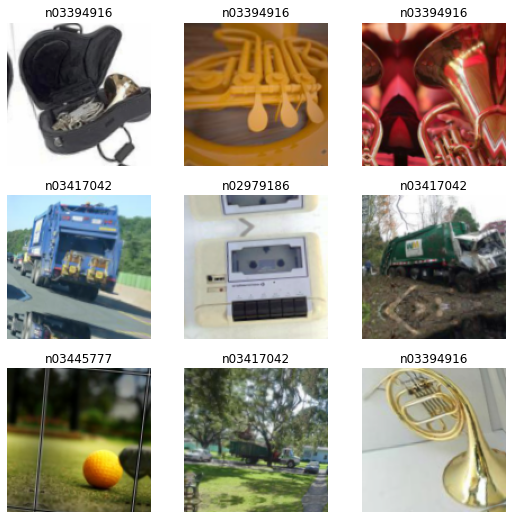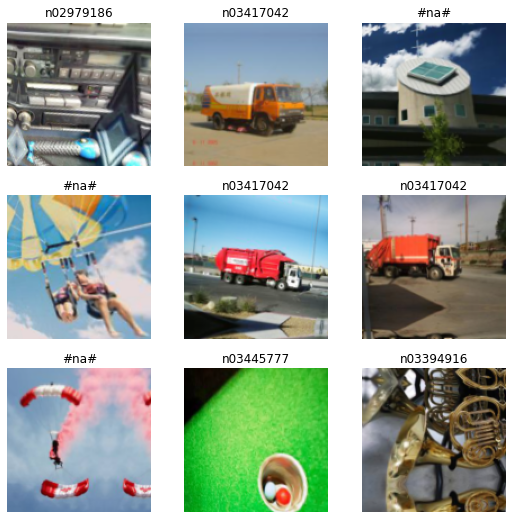Notebook contains experiments for BCENa loss, which is tweaked BCE loss that takes into account unknown and unseen before categories. Checks whether changing the target vector of an unknown category to an empty vector would be more effective than treating it the same as the others.

# Setup¶

In [ ]:
! pip install -q fastai2
! pip install -q psutil

In [ ]:
from google.colab import drive
drive.mount('/content/drive')

Drive already mounted at /content/drive; to attempt to forcibly remount, call drive.mount("/content/drive", force_remount=True).

In [ ]:
from pathlib import Path

# save models on gdrive
path = Path('/content/drive/My Drive/')
assert path.is_dir()

# save models in local folder
# path = Path('.').resolve()


# Modified loss and accuracies¶

In [ ]:
from fastai2.basics import *
from fastai2.vision.all import *
from fastai2.callback.all import *
from fastai2.distributed import *
from fastai2.optimizer import *


## accuracy metrics¶

metrics that are taking into account that first category is unknown

1. accuracy_with_na count accuracy, but all values below threshold treat as unknown caegory
2. accuracy_without_na count accuracy ommiting unknown category
In [ ]:
class SkipMetricPartException(Exception):
pass

class AvgPartMetric(AvgMetric):
"Average the values of func allowing to raise exception and omit accumulation"
def accumulate(self, learn):
try:
val, bs = self.func(learn.pred, *learn.yb)
self.total += to_detach(val)*bs
self.count += bs
except SkipMetricPartException: pass
except Exception as e: print(e)

def accuracy_with_na(inp, targ, thresh=0.4, na_idx=0, axis=-1, sigmoid=True):
"Compute accuracy assuming that prediction below threshold belongs to first category (#na#)"
if sigmoid: inp = inp.sigmoid()
valm, argm = inp.max(dim=axis)
argm[valm < thresh] = na_idx # treat all values below threshold as category #na#
inp, targ = flatten_check(argm, targ)
return (inp==targ).float().mean()

def _accuracy_without_na(inp, targ, na_idx=0, axis=-1):
"Compute accuracy with targ when pred omiting #na# category"
idxs = targ!=na_idx
if idxs.any():
inp, targ = flatten_check(inp[idxs].argmax(dim=axis), targ[idxs])
return (inp==targ).float().mean(), targ.shape[axis]
else:
# skip accumulating metric if there is only category #na# in batch
raise SkipMetricPartException

_accuracy_without_na.__name__ = 'accuracy_without_na'
accuracy_without_na = AvgPartMetric(_accuracy_without_na)


## bce na losses¶

BCENaLoss - BCE loss that is changing the target vector of an unknown category to an empty vector

In [ ]:
from fastai2.basics import *
from fastai2.vision.all import *
from fastai2.callback.all import *
from torch import nn

class BCENaLoss(nn.Module):
y_int = True

def __init__(self, logits=True, reduction='mean'):
super().__init__()
self.reduction = reduction
self.logits = logits

def forward(self, input, target):
target = F.one_hot(target, input.shape).float()
target[:, 0] = 0 # first category is #na# category so it should be zeroed
if self.logits:
return F.binary_cross_entropy_with_logits(input, target, reduction=self.reduction) # sigmoid + bce
else:
return F.binary_cross_entropy(input, target, reduction=self.reduction) # no sigmoid

@delegates(keep=True)
class BCENaLossFlat(BaseLoss):
"Same as FocalLoss, but flattens input and target."
def __init__(self, *args, axis=-1, thresh=0.5, **kwargs):
super().__init__(BCENaLoss, *args, axis=axis, **kwargs)
self.thresh = thresh

def decodes(self, x):
valm, argm = x.max(dim=self.axis)
argm[valm < self.thresh] = 0
return argm


In [ ]:
@delegates()
class BCEWithLogitsLossOneHotFlat(BCEWithLogitsLossFlat):
def __call__(self, inp, targ, **kwargs):
return super().__call__(inp, F.one_hot(targ, inp.shape), **kwargs)
def decodes(self, x):    return x.argmax(dim=-1)


Three types of categories are considered.

Known categories      - network recognises and can be returned as a prediction
Unknown categories  - may be (depends on add_na parameter) trained and recognized by network, but all falls into category #na#
Unseen categories     - are not trained and are not seen before validation

training and validation set contain known (and depends on add_na parameter) and unknown categories
test set contains always known, unknown and unseen categories

In [ ]:
def get_train_dls(bs=48, size=128, workers=None, augs=True, item_tfms=[], batch_tfms=[], add_na=False, train_na=None):
dspath = untar_data(URLs.IMAGENETTE_160)

if workers is None: workers = min(8, num_cpus()//(num_distrib() or 1))
norm_tfms = [Normalize.from_stats(*imagenet_stats)]
augs_tfms = aug_transforms() if augs else []

# categories known and trained
train_cats = [
'n03417042',
'n02979186',
'n03394916',
'n03445777',
]

# categories na and trained
na_train_cats = [
'n03028079',
'n01440764',
'n03888257',
]

# categories not trained (unseen by network)
test_cats = [
'n02102040',
'n03000684',
'n03425413'
]

if train_na is None: train_na = add_na

def get_items(dspath):
if train_na:
# train and validate known and unknown categories
train_folders = train_cats + na_train_cats
valid_folders = train_cats + na_train_cats
else:
# train and validate only known categories
train_folders = train_cats
valid_folders = train_cats

return get_image_files(dspath/'train', folders=train_folders) + get_image_files(dspath/'val', folders=valid_folders)

# create train and valid dataloaders
dbl = DataBlock(
get_items=get_items,
get_y=parent_label,
splitter=GrandparentSplitter(train_name='train', valid_name='val'),
item_tfms=item_tfms + resize_tfms,
batch_tfms=batch_tfms + augs_tfms + norm_tfms,
)

# support any unknown category as random from existing categories
# it does not affect training, only enables validation for not known categories
items = dls.valid.tfms.vocab.items
o2i = dls.valid.tfms.vocab.o2i
dls.valid.tfms.vocab.o2i = defaultdict(lambda: random.randint(0, len(items) - 1), o2i)

# add custom test dataloader to validate all cats (even unseen before)
test_items = get_image_files(dspath, folders=['val'])
dls.test = dls.test_dl(test_items, with_labels=True)

return dls


dataset with only known categories

In [ ]:
dls = get_train_dls()
dls.show_batch()dataset with known and unknown (labeled as #na#) categories

In [ ]:
dls = get_train_dls(add_na=True)
dls.show_batch()dataset with known, unknown and unseen categories

In [ ]:
dls.test.show_batch()